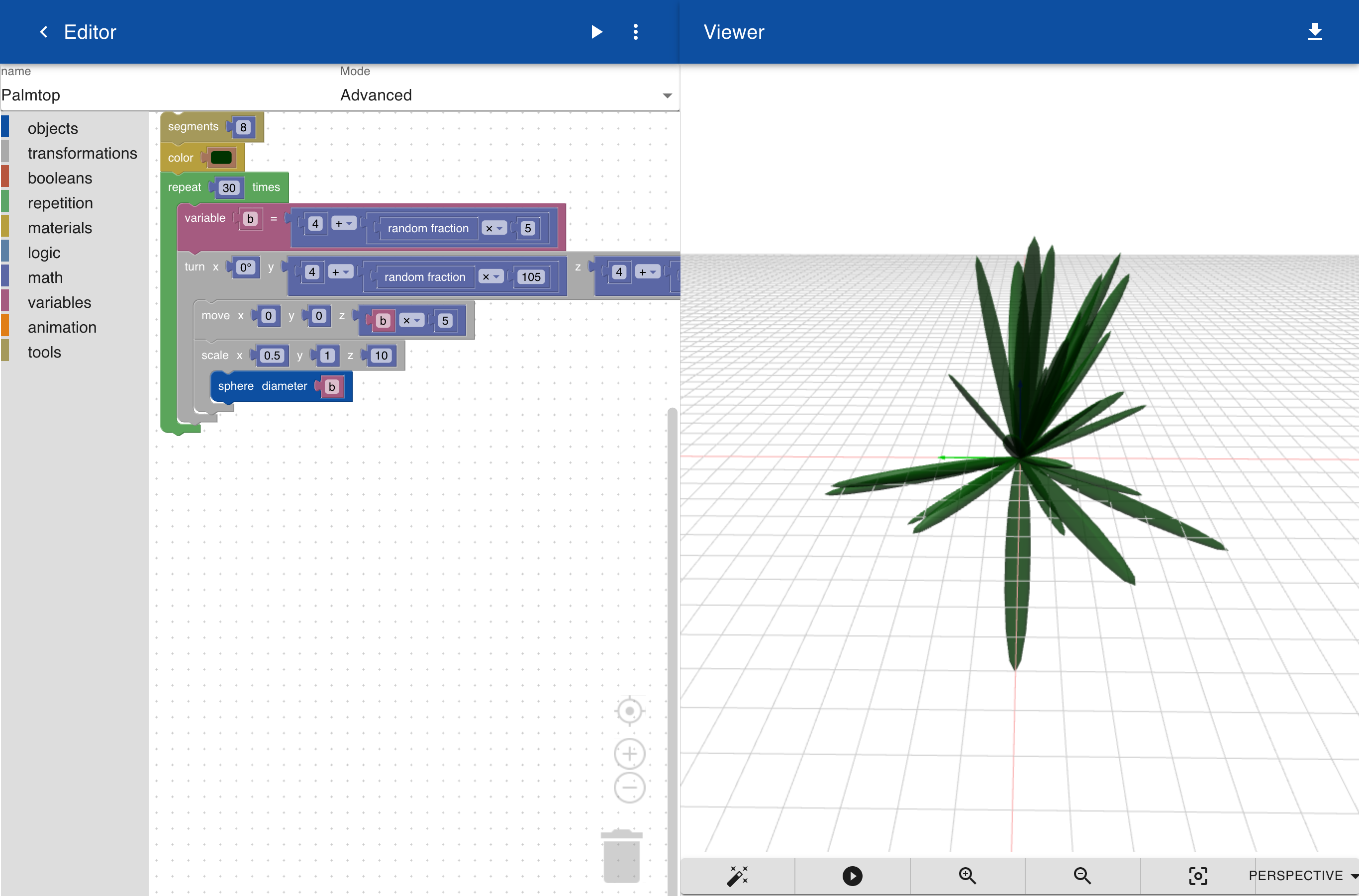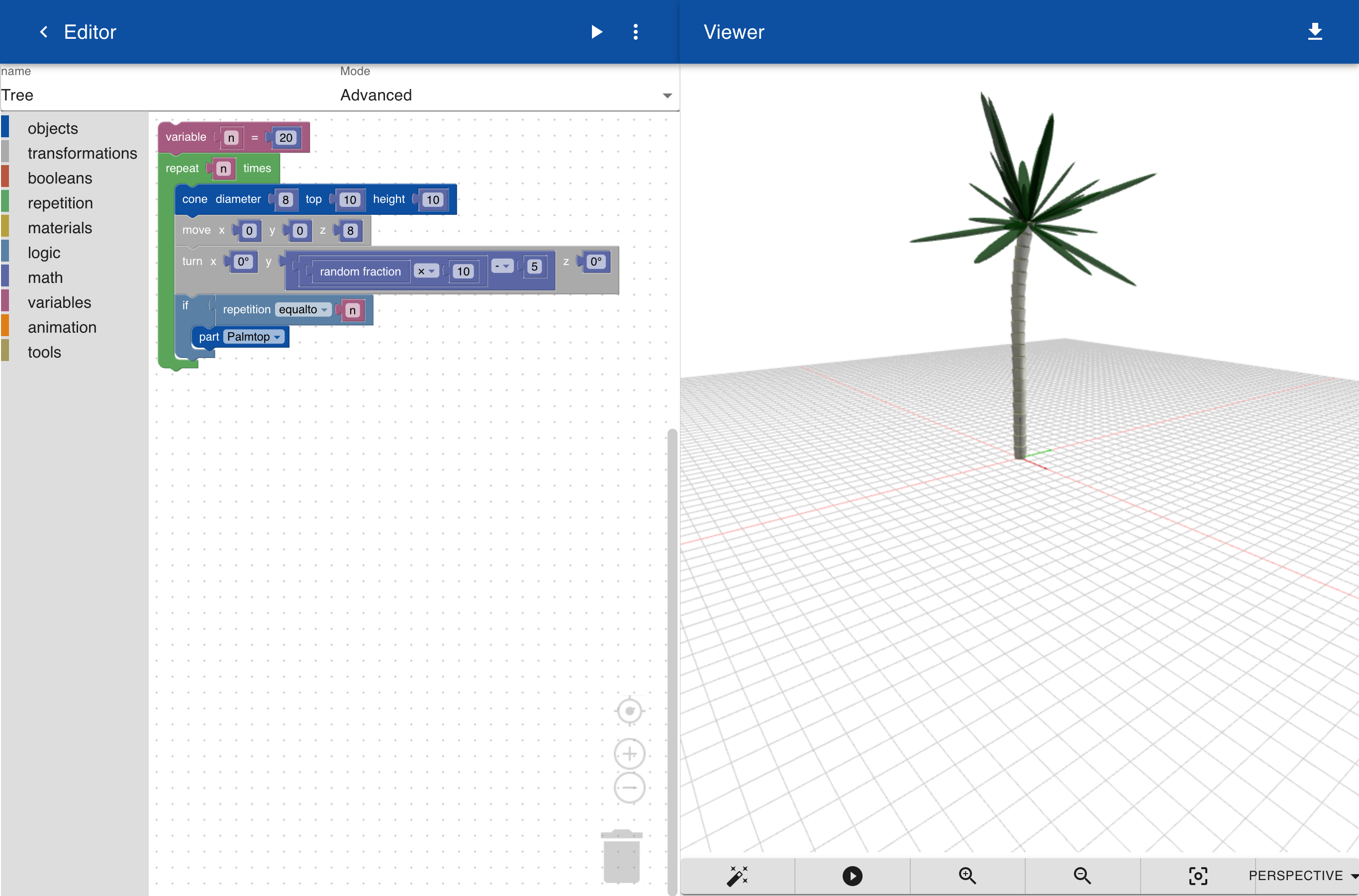In addition to `parameter`, which defines the variables for a part, you can also use `variable`. A variable is only used inside a part and are not exposed outside. Parameters are similar to arguments in functions in programming languages and variables are internal variables. In the object below we define a variable `b` to set the size of a leaf. The variable is defined by an expression that includes a `random fraction` function, wich can be found under the `math` menu. A random fraction is a number between `0` and `1` like `0.12345`. The random fraction is multiplied by 5 and then 4 is added. This means that the size of the leaf, variable `b` will always between `4` and `9`. The leaf itself is create from a sphere that is scaled `0.5` in X-direction to make it a bit flat. It is scaled in Z-direction to make it long. Another random expression is used to set a random turn around the Y-axis and Z-axis.The `Palmtop` object is included in a tree. The tree consists of a number of cones that are randomly rotated a between `-5` and `5` degrees. Note that every time that you generate the tree it will look a bit different.